Dec. 3, 2019

# Diagnose like a Radiologist. Attention-based model on TensorFlow 2.0.

In the last few years, the attention mechanism has become a popular technique to improve the accuracy of neural networks, we will see what this mechanism is and how to implement the model from the paper. To implement this model we will use TensorFlow 2.0. This new version of TensorFlow is really good when we have to do multiple actions to train our models.

## Attention mechanism

Some months ago I wrote a post about class activation maps, this technique shows us the most important areas of the input image that the neural network took into account to make the final prediction. We obtain these activation maps from the output feature maps of the layers of the neural network.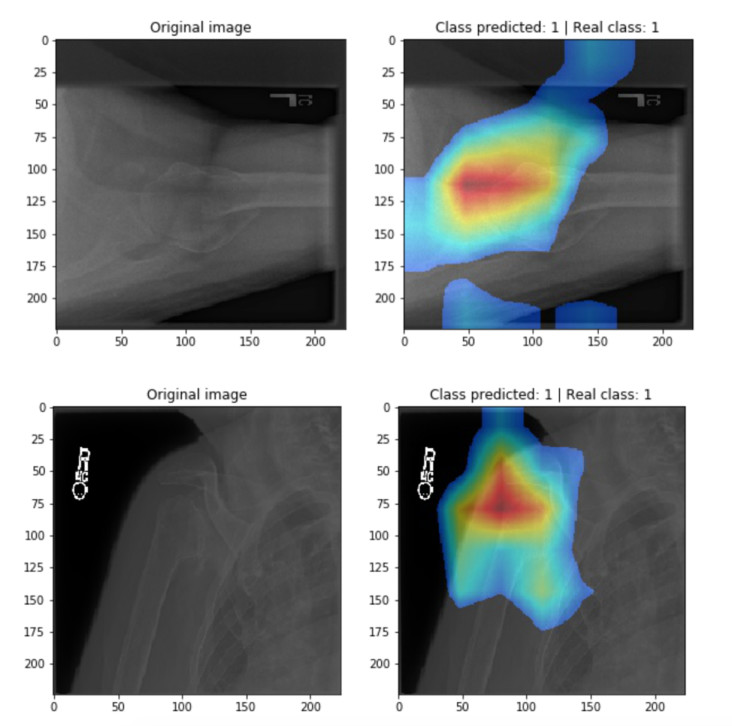Activation maps. Image from the class activation maps post.

There are multiple ways to implement an attention mechanism, in fact, this technique comes from NLP models where we want the model to pay attention to the most important words in a phrase. We can use a trainable attention mechanism where the mechanism is trained while the network is trained, like in the Learn To Pay Attention paper where this mechanism is integrated inside the architecture and affects directly the network's outputs.

We can also use a trained model to obtain the activation maps and crop the most important area of the images and train a new model on these cropped images. This was the approach that the authors of the [Diagnose like a Radiologist: Attention Guided

Convolutional Neural Network for Thorax Disease

Classification](https://arxiv.org/abs/1801.09927) paper followed. The attention mechanism follows this idea of getting the activation maps from the layers of the neural network and use them to learn where the network should pay attention to improve the training and accuracy of the predictions.

The authors of the Diagnose like a Radiologist paper implemented a three-branch neural network, each branch is a convolutional neural network that resolves a multi-label classification problem. One of these networks presents the attention mechanism but before talking about these models we must know the problem that the paper is trying to solve.

## Thorax Disease classification

In this paper the authors used the ChestX-ray14 dataset that contains 112,120 frontal-view chest X-ray images of 30,805 unique patients, each image is labeled with one or more types of 14 thorax diseases. In total, we have 15 classes, where the first class indicates if the patient is healthy or not.

Thorax diseases can happen in small localized areas, if we use the whole image to train a model, the model accuracy could be affected by the irrelevant areas of the image. However, some diseases like pneumonia have lesion areas distributed in the whole image, therefore we should take into account small and big lesion areas to perform a final prediction. To solve this problem, the authors used three models, a global model, a local model, and a fusion model.

## Three-branch neural network

These models have the same aim, to predict correctly the diseases of the image, the global model takes as input the whole x-ray image from the ChestX-ray14 dataset that we call global image, the local model takes as input an image that we call local image, we obtain the local image using the activation maps from the global model, as I previously mentioned, these activation maps show the most important area of the image, and in this case the area contains the lesion of the disease. Due to this, the local model introduces the attention mechanism and the model can focus on the lesion area in a better way.

If we have a small lesion area the local model will perform better than the global model since the global model has to deal with irrelevant areas, whereas, if the lesion area is distributed in the whole image the local model is not good enough to make a good prediction, here the global model will perform better, for this reason, the fusion model combines the learning of these two models to perform a final strong prediction.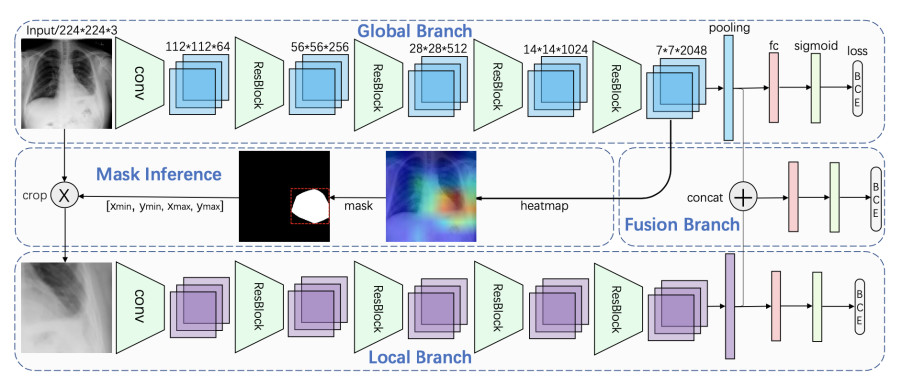Three-branch neural network. Image from the Diagnose like a Radiologist: Attention Guided Convolutional Neural Network for Thorax Disease Classification paper.

We will check one by one these models and their architectures. I recommend you to follow this Jupyter Notebook to understand better the implementation.

### Global Model

The global model uses as base network the ResNet50 or DenseNet121 architectures. In this case, we will implement the network using the DenseNet121 architecture since it's faster to train.

The following code is the implementation of this network:

``````
def create_branch():

dense_model = DenseNet121(

weights="imagenet",

input_shape=image_size,

include_top=False)

feature_maps = dense_model.output

pooling_layer = layers.GlobalMaxPool2D()(feature_maps)

predictions = layers.Dense(num_classes, activation="sigmoid")(pooling_layer)

model = Model(inputs=dense_model.input, outputs=[predictions, feature_maps, pooling_layer])

weight_decay_value = 1e-4

weight_decay = tf.keras.regularizers.l2(l=weight_decay_value)

for layer in model.layers:

layer.trainable = True

if hasattr(layer, 'kernel_regularizer'):

layer.kernel_regularizer = weight_decay

return model

``````

Here, we can notice that we use the dense network pre-trained on the imagenet dataset and our model has multiple outputs, these outputs are:

• predictions: These are the predictions for each class. Since we have a multi-label classification problem we use the sigmoid function so each class has a value between 0 and 1 and the output is an array like [0, 0.45, 0.78, 0.23, 0.98].

• feature_maps: These are the output feature maps of the last layer of the DenseNet model, we use these feature maps to get the activation maps and crop the global image to obtain the local image.

• pooling_layer: This pooling layer is used to train the fusion model and get a prediction based on the decisions of the global and local models.

Finally, we use L2 or weight decay regularization in each convolutional layer to avoid overfitting.

### Local Model

The local model uses the same architecture than the global model but these two models don't share weights and are trained on different iterations. We use the same create_branch function to create the global and local models, however, in the local model we ignore the feature maps since we don't need them.

### Fusion model

The fusion model takes as input the last pooling layers of the global and local models:

``````
def create_fusion_branch():

global_pool = layers.Input(shape=(1024))

local_pool = layers.Input(shape=(1024))

concat_layer = layers.concatenate([global_pool, local_pool])

model = layers.Dense(num_classes, activation="sigmoid")(concat_layer)

model = Model(inputs=[global_pool, local_pool], outputs=model)

return model

``````

We concatenate these pooling layers and use a fully connected layer to obtain the predictions.

## Training

To train these models we use the binary crossentropy loss function and the area under the curve metric:

``````
def compute_loss(true_classes, pred_classes):

loss = tf.keras.losses.binary_crossentropy(true_classes, pred_classes)

return tf.reduce_mean(loss)

``````
``````
auc_metric = tf.keras.metrics.AUC()

def compute_metrics(true_classes, pred_classes):

auc_metric.reset_states()

auc_metric.update_state(true_classes, pred_classes)

auc_value = auc_metric.result()

return auc_value

``````

We train each model in three stages:

### Stage 1

Firstly, we train the global model using a batch size of 64:

``````
@tf.function

def train_stage_1(batch_images, labels):

with tf.GradientTape() as tape:

pred_classes, _, _ = global_branch_model(batch_images, training=True)

loss = compute_loss(labels, pred_classes)

auc_value = compute_metrics(labels, pred_classes)

return loss, auc_value

``````

The function above is computed for each batch multiples times during one epoch. This function updates the model's weights.

``````
def train(epochs):

for epoch in range(epochs):

batch_time = time.time()

step = 1

for batch_images, labels in train_generator:

loss, auc_value = train_stage_1(batch_images, labels)

auc_value = auc_value.numpy()

loss = loss.numpy()

print('\r', 'Epoch', f"{epoch + 1}/{epochs}", '| Step', f"{step}/{train_steps}",  '| loss:', loss, "| AUC:", auc_value, "| time:", time.time() - batch_time, end='')

step += 1

stage_1_loss_results.append(loss)

stage_1_auc_results.append(auc_value)

batch_time = time.time()

checkpoint.save(file_prefix=checkpoint_prefix)

if (epoch + 1) % 20 == 0:

current_lr = tf.keras.backend.get_value(global_branch_optimizer.lr)

new_lr = current_lr / 10

tf.keras.backend.set_value(global_branch_optimizer.lr, new_lr)

print('\r', 'Epoch', f"{epoch + 1}/{epochs}", '| Step', f"{step}/{train_steps}",  '| loss:', loss, "| AUC:", auc_value, "| time:", time.time() - start)

``````

The train function executes the train_stage_1 function multiple times to train the model, this function prints the loss and the auc values after each batch, also after 20 epochs, we decrease the learning rate value as the paper mentions.

### Stage 2

Once we have the global model trained, we use it to get the local images and train the local model on these images. In this step we only train the local model and we don't update the global model's weights.

#### Local Images

To get the local images, we need a function to compute the activation maps of the global model and crop the lesion area of the global images:

``````
def get_local_images(feature_maps, global_images):

batch_size = len(feature_maps)

local_images = np.zeros((batch_size, 224, 224, 3), dtype=np.float32)

for index in range(batch_size):

feature_map = feature_maps[index, ...]

global_image = global_images[index, ...]

l1_distance = tf.norm(feature_map, ord=1, axis=2) # outputs 7x7

l1_distance = l1_distance - tf.keras.backend.min(l1_distance)

heatmap = l1_distance / tf.keras.backend.max(l1_distance)

resized_heatmap = tf.image.resize(tf.expand_dims(heatmap, 2), image_size[0:2], method=tf.image.ResizeMethod.BILINEAR)

binary_mask = tf.where(resized_heatmap[..., 0] > treshhold, 1, 0)

labeled_mask, labels_indexes = label(binary_mask, connectivity=2, background=0, return_num=True)

elements_by_label = [np.sum(labeled_mask == label_index) for label_index in range(1, labels_indexes + 1)]

if len(elements_by_label) == 0:

max_connected_region = (labeled_mask == -1)

else:

max_label_index = np.argmax(elements_by_label) + 1

max_connected_region = (labeled_mask == max_label_index)

max_connected_region_stack = tf.stack([max_connected_region, max_connected_region, max_connected_region], axis=2)

masked_image = tf.cast(max_connected_region_stack, tf.float32) * global_image

min_y = min(mask_coords[:, 0])

min_x = min(mask_coords[:, 1])

max_y = max(mask_coords[:, 0])

max_x = max(mask_coords[:, 1])

local_image = global_image[min_y:max_y, min_x:max_x, :]

local_image = tf.image.resize(local_image, image_size[0:2], method=tf.image.ResizeMethod.BILINEAR)

local_images[index, ...] = local_image

return local_images

``````

The function get_local_images will use the feature maps to compute the attention maps and crop the global images. Since we have multiple images we need a for loop.

In the paper, I found this part a little bit confusing so I searched how to implement the attention maps and I found out a pythorch implementation. The get_local_images function is a similar implementation but using TensorFlow.

To train the local model, we use a batch size of 32, therefore we need 32 feature maps and 32 global images. We will compute the attention maps one by one:

``````
feature_map = feature_maps[index, ...]

global_image = global_images[index, ...]

``````

The paper indicates that we can compute the l1 distance of the feature maps and then count the maximum values to get a heatmap:

``````
l1_distance = tf.norm(feature_map, ord=1, axis=2)

l1_distance = l1_distance - tf.keras.backend.min(l1_distance)

heatmap = l1_distance / tf.keras.backend.max(l1_distance)

``````

The size of each feature map is 7x7x1024, we compute the l1 distance across the channel axis (axis 2) and we end up with a feature map of size 7x7, then we subtract the minimum value of this feature map to the feature map itself, I am not sure about the reason behind the subtraction, however, if we do this we obtain a better heatmap. Finally, we divide the feature map by the maximum value to count the maximum values of the feature map to get our heatmap. We can see this heatmap as a grayscale image where each pixel has values between 0 and 1.

Our heatmap has a size of 7x7, we have to resize this heatmap to the same size as our input image (224, 224), we use a bilinear method where each new pixel will have a similar value than the near pixels but not the same.

``````
resized_heatmap = tf.image.resize(tf.expand_dims(heatmap, 2), image_size[0:2], method=tf.image.ResizeMethod.BILINEAR)

``````

We will compute a binary mask from our heatmap. If some pixel has a value greater than 0.7 we assign 1 to that pixel, otherwise we assign 0.

``````
binary_mask = tf.where(resized_heatmap[..., 0] > treshhold, 1, 0)

``````

The final binary mask looks like: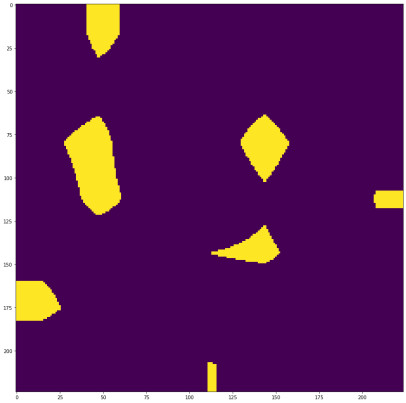Where the yellow areas are ones and the purple areas are zeros.

To get the lesion area, we have to find out the maximum connected region of this binary mask, in other words we have to find out the biggest yellow area. To achieve this we will use the label function from skimage that labels connected pixels:

``````
labeled_mask, labels_indexes = label(binary_mask, connectivity=2, background=0, return_num=True)

``````

Here the label function considers the zero values as background and the connectivity indicates that a pixel must have at least 2 pixels close to another pixel to consider them of the same label. This function returns the number of areas labeled and the following labeled_mask where we can notice that now each area has a different color: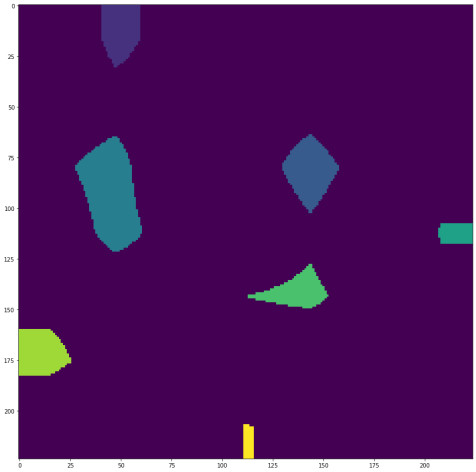Now we have to count the number of pixels that each area has and, the label with more pixels is the maximum connected region:

``````
elements_by_label = [np.sum(labeled_mask == label_index) for label_index in range(1, labels_indexes + 1)]

``````

We also have to check if the mask was labeled or if the label function couldn't find any connected region:

``````
if len(elements_by_label) == 0:

max_connected_region = (labeled_mask == -1)

else:

max_label_index = np.argmax(elements_by_label) + 1

max_connected_region = (labeled_mask == max_label_index)

``````

If the mask is labeled, then we keep the maximum connected region: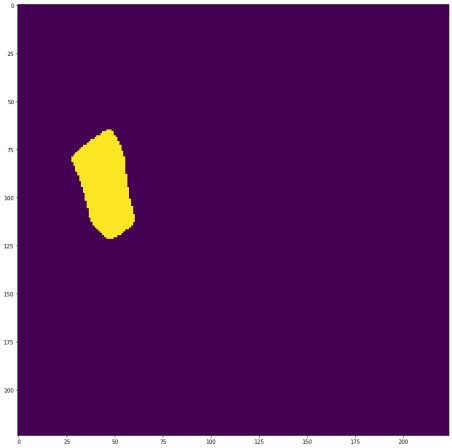maximum connected region:

This yellow area is the maximum connected region that contains the lesion area. If we multiply the global image by this connected region, we only keep the pixels of the image that belong to the lesion area:

``````
max_connected_region_stack = tf.stack([max_connected_region, max_connected_region, max_connected_region], axis=2)

masked_image = tf.cast(max_connected_region_stack, tf.float32) * global_image

``````

However, we need a square area to crop the global image correctly. For this reason, we need to find out the coordinates where the pixels are not zero:

``````

``````

`np.argwhere` returns the indices of the elements that are non-zero, using these indices we find the minimum values to get the min_y and min_x coordinates and the maximum values to get the max_y and max_x coordinates:

``````
min_y = min(mask_coords[:, 0])

min_x = min(mask_coords[:, 1])

max_y = max(mask_coords[:, 0])

max_x = max(mask_coords[:, 1])

``````

With these coordinates, now we can crop the global image to obtain the local image:

``````
local_image = global_image[min_y:max_y, min_x:max_x, :]

``````

Finally, we have to resize the local image to the input size of the network (224, 224, 3):

``````
local_image = tf.image.resize(cropped_image, image_size[0:2], method=tf.image.ResizeMethod.BILINEAR)

``````

In the example above, I used a random numpy array as feature maps to show how the function works. However, if we use real feature maps from the global model, the heatmap and binary mask are a little bit different: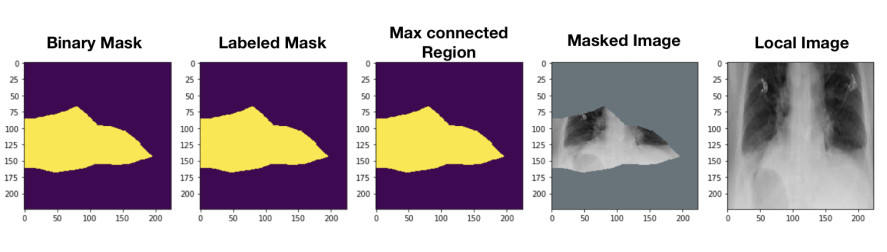In this case, the binary mask and labeled mask are the same since we only have one connected region and, therefore, one label..

The input of the get_local_images function are the feature maps from the global model and global images like the following: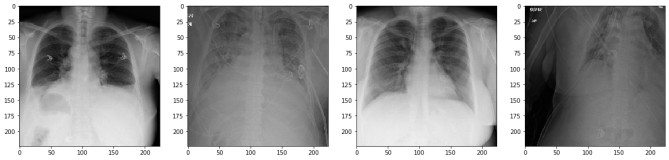And the output are the local images: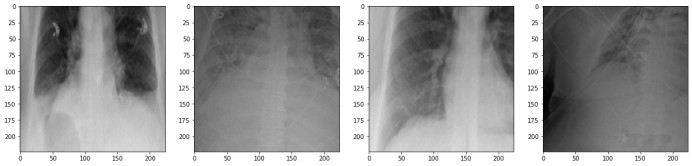We can notice how the local images focus on some areas of the global image. I have to mention that I used a global model trained only for one epoch to obtain these local images, a model trained for more epochs should do a better job localizing the lesion areas.

#### Training Local Model

The following function is quite similar than the function we previously used to train the global model each batch:

``````
@tf.function

def train_stage_2(local_images, labels):

with tf.GradientTape() as tape:

pred_classes, _, _ = local_branch_model(local_images, training=True)

loss = compute_loss(labels, pred_classes)

auc_value = compute_metrics(labels, pred_classes)

return loss, auc_value

``````

The function to train our model each epoch is the following one:

``````
def train(epochs):

for epoch in range(epochs):

batch_time = time.time()

epoch_time = time.time()

step = 1

for batch_images, labels in train_generator:

_, feature_maps, _ = global_branch_model(batch_images, training=False)

local_images = get_local_images(feature_maps, batch_images)

loss, auc_value = train_stage_2(local_images, labels)

auc_value = auc_value.numpy()

loss = loss.numpy()

print('\r', 'Epoch', f"{epoch + 1}/{epochs}", '| Step', f"{step}/{train_steps}",  '| loss:', loss, "| AUC:", auc_value, "| time:", time.time() - batch_time, end='')

step += 1

stage_2_loss_results.append(loss)

stage_2_auc_results.append(auc_value)

batch_time = time.time()

if (epoch + 1) % 20 == 0:

current_lr = tf.keras.backend.get_value(local_branch_optimizer.lr)

new_lr = current_lr / 10

tf.keras.backend.set_value(local_branch_optimizer.lr, new_lr)

checkpoint_stage2.save(file_prefix=checkpoint_stage2_prefix)

print('\r', 'Epoch', f"{epoch + 1}/{epochs}", '| Step', f"{step}/{train_steps}",  '| loss:', loss, "| AUC:", auc_value, "| time:", time.time() - epoch_time)

``````

Here we use the feature maps from the global model (global_branch_model) to obtain the local images (get_local_images). Finally, we use these local images to train the local network (train_stage_2).

### Stage 3

Now that we have the local and global models trained, we can train the fusion model:

``````
@tf.function

def train_stage_3(global_pooling_layers, local_pooling_layers, labels):

with tf.GradientTape() as tape:

pred_classes = fusion_branch_model([global_pooling_layers, local_pooling_layers], training=True)

loss = compute_loss(labels, pred_classes)

auc_value = compute_metrics(labels, pred_classes)

return loss, auc_value

``````

This model takes as input the pooling layers of the previous models and not the images.

``````
def train(epochs):

for epoch in range(epochs):

batch_time = time.time()

epoch_time = time.time()

step = 1

for batch_images, labels in train_generator:

_, feature_maps, global_pooling_layers = global_branch_model(batch_images, training=False)

local_images = get_local_images(feature_maps, batch_images)

_, _, local_pooling_layers = local_branch_model(local_images, training=False)

loss, auc_value = train_stage_3(global_pooling_layers, local_pooling_layers, labels)

auc_value = auc_value.numpy()

loss = loss.numpy()

print('\r', 'Epoch', f"{epoch + 1}/{epochs}", '| Step', f"{step}/{train_steps}",  '| loss:', loss, "| AUC:", auc_value, "| time:", time.time() - batch_time, end='')

step += 1

stage_3_loss_results.append(loss)

stage_3_auc_results.append(auc_value)

batch_time = time.time()

if (epoch + 1) % 20 == 0:

current_lr = tf.keras.backend.get_value(fusion_branch_optimizer.lr)

new_lr = current_lr / 10

tf.keras.backend.set_value(fusion_branch_optimizer.lr, new_lr)

checkpoint_stage3.save(file_prefix=checkpoint_stage3_prefix)

print('\r', 'Epoch', f"{epoch + 1}/{epochs}", '| Step', f"{step}/{train_steps}",  '| loss:', loss, "| AUC:", auc_value, "| time:", time.time() - epoch_time)

``````

We can see that we are using the global and local models to get the pooling layers and, at the same time, we are using the global model to get the local images.

## The Notebook

In this post, we have seen some parts of the code to create the models and train them. However, I recommend checking the notebook to review the whole process. In the notebook, there is the code to get the dataset and create the generators.

If you want to test the get_local_images function or print the images using a tool like matplotlib then you must use the following code to see the images correctly:

``````
imagenet_mean = np.array([103.939, 116.779, 123.68])

image = tf.math.add(image, imagenet_mean) / 255.0

``````

I couldn't train the models on Google Colab since the process was taking a lot of time. I hope this code is useful if you want to implement this architecture and also if you want to check TensorFlow 2.0 and its workflow.

## Identity Confounding

Before ending this post, I would like to talk about one more thing. Perhaps you have seen or read that medical data is not good enough to train machine learning or deep learning models. I am not an expert in this field but, I know that if we train models to diagnose diseases, we should be very careful since there are lives depending on the decisions of our model. Sometimes, we can get a really good accuracy value or even good sensitivity and specificity values, which are better metrics if our dataset is not balanced. However, the quality of our models depends in a big way on the quality of the dataset.

The authors of this paper used the ChestX-ray14 dataset, this paper is from January 2018 and the dataset is a little bit older, ChestX-ray14 is a dataset that contains incorrect labels for a lot of images, even when the average area under the curve across the 14 diseases is 87.1 on the test set, we can not say that this model is good to use in real-life environments. This good test set result is due to the consistent of the data, both sets are wrongly labeled, the model can still fit the data but in production, the predictions are not useful.

To train these models the authors split the dataset into training, validation and test sets randomly. Therefore, the x-rays of a patient can appear in multiple sets, this introduces a problem called Identity Confounding where the model learns to identify the patients instead of the disease since the first tasks is easier than the last one, when we test the model on the validation or test sets the model that learned to identify patients, sees the same patient and knows if has some disease or not instead of identifying the disease from the image.

Identity Confounding is not the only problem that we can find in medical images, several problems can fool us and make us think that our model is good enough. These problems also cause difficulties to human doctors, to build a good dataset and a good model, researches must work along with human doctors to understand the workflow of the latter.

In this paper the authors replicate the workflow that radiologists follow, first the radiologist checks the whole image area to discover the lesion, once the lesion area has been identified, the radiologist will concentrate on this area, finally, the radiologist will consider both areas to make a final prediction. The idea of mimic the workflow of real doctors is really good to implement new architectures.